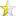Feb 19, 2008 (last update: Feb 19, 2008)

# Dynamic allocation in C/C++WHAT IT IS
----------

Dynamic allocation is the automatic allocation of memory in C/C++, Unlike declarations, which load data onto the programs data segment, dynamic allocation creates new usable space on the programs STACK (an area of RAM specifically allocated to that program).

It is accomplished by two functions (in C) and two operators (in C++):
malloc and free, new and delete.

WHY YOU USE IT
--------------

Dynamic allocation can be quite useful. For example, a dynamically sized array:

Assume you have variable size as a variable (it is NOT defined as constant). The compiler will give you an error if you try this:
BOTH VERSIONS
 `` `` ``int array[size];``

What you can do is allocate this memory dynamically. For example:
C VERSION:
 ``12`` ``````int *array; array=(int *) malloc(size*sizeof(int));``````

C++ VERSION:
 ``12`` ``````int *array; array=new int[size]l``````

NOTICE:
Since this data is pushed on the stack, not the segment, a possibility of MEMORY LEAKS occurs. Memory leaks are where allocated memory is not freed before the program returns. For example, to safely deallocate the array just created (and normally return from the program):
C VERSION:
 `` `` ``free(array);``

C++ VERSION:
 `` `` ``delete [] array``

Another special notice: the brackets immediately following delete are only necessary if you allocated an array originally.

Dynamic allocation is NECESSARY to there use of linked lists. Assuming self-referential structure Node has been declared with nextptr being the link:
C VERSION:
 ``1234567`` ``````struct Node *newptr; newptr=(struct Node *) malloc(sizeof(Node)); /*We'll insert this into this linked list just at the second item, assuming topptr is the name of the top of this list.*/ newptr->nextptr=topptr->nextptr; topptr->nextptr=newptr;``````

C++ VERSION:
 ``12345`` ``````Node *newptr; newptr=new Node; //We'll insert this as the second item, using topptr. newptr->nextptr=topptr->nextptr; topptr->nextptr=newptr;``````

Of course, in both of these examples, no other members within structure Node are used, which defeats the purpose of this linked list. To free a node:
C VERSION:
 ``1234567`` ``````struct Node *tempptr; /*The node we'll free is, again, the second.*/ tempptr=topptr->nextptr; /*We'll unbind this node first, so as not to lose all of the rest of the list with it.*/ topptr->nextptr=tempptr->nextptr; /*It's free, get rid of it.*/ free(tempptr);``````

C++ VERSION:
 ``1234567`` ``````Node *tempptr; //We'll get the second node again. tempptr=topptr->nextptr; //Unbind this node. topptr->nextptr=tempptr->nextptr; //Get rid of it. delete tempptr;``````

HOW TO USE IT
-------------

The syntax, for the C functions, is:
 ``123`` ``````#include void *malloc(size_t size)``````

The casting in the previous examples is necessary because malloc returns a void pointer.
 ``123`` ``````#include void free(void *);``````

This doesn't need casting; anything passed is implicitly demoted.

The operators in C++ are:
 `` `` ``NewAlloc=new Type;``

C++ will automatically cast for you.
 ``1234`` `````` //If this is an array delete [] NewAlloc; //Otherwise delete NewAlloc;``````

GOING FARTHER
-------------

Several classes, like vector and list, use this. Implement your own!

Understanding this article requires a good understanding of pointers and arrays (and their relationships). I know, I should put this at the top.

Define a class (I named mine SafeSpot) that will automatically handle the deallocation of memory defined within it when the object is destroyed. This will ELIMINATE all of those unforseen memory leaks. You may want to try different scopes of this class for different scopes of allocation (don't let a temp function object exist for the whole program).